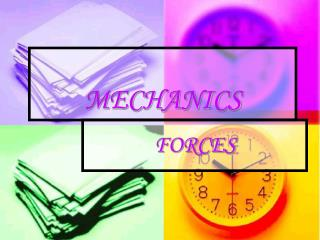# MECHANICS - PowerPoint PPT PresentationDownload PresentationMECHANICS

MECHANICSDownload Presentation## MECHANICS

- - - - - - - - - - - - - - - - - - - - - - - - - - - E N D - - - - - - - - - - - - - - - - - - - - - - - - - - -
##### Presentation Transcript

1. MECHANICS FORCES

2. Force Forces can: • Change the speed of an object • Change the direction of movement of an object • Change the shape or size of an object

3. Electric Forces: These exist between electric charges, or between electric charges or between electric fields. Magnetic forces: These exist between magnets and currents. Nuclear Forces: These forces are very strong, they hold together particles in molecules. Gravitational Forces: These act between masses. They are weak but may become sizeable if one of the masses is big. Nuclear Forces: These are very strong forces, they hold particles together in the nucleus. Gravitational forces: These act between masses. Non Contact Forces

4. Weight Weight is a very common force. Weight is the force gravity due to the pull of the earth, this is the force that is pulling downwards towards the centre of the earth. W=mg W-weight g-gravity=10Nkg-2 Nkg-2=ms-2

5. The turning effects of Forces • When forces act at different points on objects the make the, turn or they may not. E.g. Using a spanner to undo a nut that is tightly done up the job becomes easier , The greater the force , and the further from the nut it can be applied, the greater is the turning effect.

6. Moments of a Force The moment o f a force is defined as the product of the force and the perpendicular distance of the turning point (fulcrum) to the line of action of the force. A moment is described as clockwise or anticlockwise depending on the direction of it turning effect. Moment=force x distance Units of moment:Nm

7. The Principle Of Moments When an object is in equilibrium, the sum of the anticlockwise moments about any point is equal to the sum of the clockwise moments about that point.

8. Levers Levers are force multipliers. In a lever an effort is applied to overcome a resistive force called a load. Example of Levers: • Scissors • Pliers • Wheelbarrow • Hammer

9. Centre or Gravity The centre of gravity of a body is the single point at which the entire weight of a body can be considered to act.

10. Irregular objects Use the plumb line method Regular objects Even no. of vertices: draw diagonal lines from one vertices to another and their intersection is the c.o.g. Methods for determining the centre of gravity

11. Equilibrium Equilibrium is a stat in which all variables are equal. There are 3 types of equilibrium: • Stable –when a body is slightly displaced the c.o.g rises and moves closer to the pivoted end. • Unstable-when a body is displaced even further its c.o.g falls . • Neutral-the c.o.g remains unchanged in its new position.

12. General F/e graph showing Hooke’s law

13. Hooke’s Law Hooke’s law states that the applied force to spring is directly proportional to the extension produced provided that the spring does not exceed its elastic limit. The graph representing this is a straight line graph when a curve is seen on the graph this means that the elastic limit has been exceeded an the spring is now deformed. Force is proportional to extension. F=ke where k is the constant.